Foxtable(狐表)用户栏目专家坐堂 → 增加一行数据的代码，执行无效

共有924人关注过本帖树形打印复制链接

# 主题：增加一行数据的代码，执行无效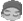edisontsui
1楼 | 信息 | 搜索 | 邮箱 | 主页 | UC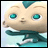加好友发短信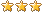If e.DataCol.Name = "出库数量" Then    Dim dr221 As DataRow = DataTables("批号表").Find("物料编号 = " & "'" & e.DataRow("物料编号") & "' and 批号 = '" & e.DataRow("批号") & "'  and 库位代号 = '" & e.DataRow("库位代号") & "' ")     If dr221 IsNot Nothing Then        If e.newvalue > dr221("库存数量") Then             e.DataRow("出库数量") = dr221("库存数量")            Dim dra As Row = Tables("出入库").AddNew()            dra("出入库单号") = e.DataRow("出入库单号")            dra("指采销单号") = e.DataRow("指采销单号")            dra("指采销行号") = e.DataRow("指采销行号")            dra("物料编号") = e.DataRow("物料编号")            dra("指采销行号") = e.DataRow("指采销行号")            dra("数量") = e.newvalue - dr221("库存数量")         End If    End IfEnd If上面代码的目的是：当在“出入库”这个表的“出库数量”一列发生变化时（比如是2000），就会去“批号表”那里找同物料编号、同批号、同库位代号的物料，看看其“库存数量”是多少（比如有1500）。此时，库存不够出货，当前“出库数量”就会自动变为1500，同时在“出入库”里面自动新增一行数据，把剩余的500个无法出货的数据填入新增这行来。但是，现在执行起来，新增行的代码无效，估计是新增行时无法记住尚未出货的500这个数据。请问要怎样修改代码呢？谢谢。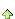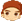2楼 | 信息 | 搜索 | 邮箱 | 主页 | UC加好友发短信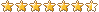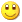Post By：2022/8/13 16:21:00 [只看该作者]

 If dr221 IsNot Nothing Then        If e.newvalue > dr221("库存数量") Then dim n as double = e.newvalue - dr221("库存数量")            e.DataRow("出库数量") = dr221("库存数量")            Dim dra As Row = Tables("出入库").AddNew()……            dra("数量") = n        End If    End IfEnd Ifedisontsui
3楼 | 信息 | 搜索 | 邮箱 | 主页 | UC加好友发短信Post By：2022/8/15 8:46:00 [只看该作者]

 If e.DataCol.Name = "出库数量" Then    Dim dr221 As DataRow = DataTables("批号表").Find("物料编号 = " & "'" & e.DataRow("物料编号") & "' and 批号 = '" & e.DataRow("批号") & "'  and 库位代号 = '" & e.DataRow("库位代号") & "' ")     If dr221 IsNot Nothing Then        If e.newvalue * (-1) > dr221("库存数量") Then             Dim q1 As Double = e.newvalue (-1) - dr221("库存数量")            e.DataRow("出库数量") = dr221("库存数量") * (-1)            Dim dra As Row = Tables("出入库").AddNew()            dra("出入库单号") = e.DataRow("出入库单号")            dra("指采销单号") = e.DataRow("指采销单号")            dra("指采销行号") = e.DataRow("指采销行号")            dra("物料编号") = e.DataRow("物料编号")            dra("指采销行号") = e.DataRow("指采销行号")            dra("出库数量") = q1        End If    End IfEnd If上述2楼的修改我明白意思了。但是，(1) 增加行的代码不起作用，也就是说不会新增加行; (2) 上面红色那句不起作用，新的出库数量还是 e.newvalue 那个数，而不是 dr221("库存数量")。顺便说一下，我们"出入库"这个表的出库数量用负数表示的。谢谢。4楼 | 信息 | 搜索 | 邮箱 | 主页 | UC加好友发短信Post By：2022/8/15 9:00:00 [只看该作者]

 调试msgbox(dr221 IsNot Nothing )    If dr221 IsNot Nothing Thenmsgbox("e.newvalue=" & e.newvalue )msgbox("库存数量=" & dr221("库存数量") )        If e.newvalue * (-1) > dr221("库存数量") Then msgbox(1)            Dim q1 As Double = e.newvalue * (-1) - dr221("库存数量")msgbox("q1 =" & q1 )            e.DataRow("出库数量") = dr221("库存数量") * (-1)msgbox("出库数量 =" & e.DataRow("出库数量"))            Dim dra As Row = Tables("出入库").AddNew()msgbox(2)            dra("出入库单号") = e.DataRow("出入库单号")edisontsui
5楼 | 信息 | 搜索 | 邮箱 | 主页 | UC加好友发短信Post By：2022/8/15 15:01:00 [只看该作者]

 Dim Filter3 As String = "[物料编号] = '" & ctn(s-1) & "'"            Dim drs3 As List(Of DataRow) = DataTables("出入库2").Select(Filter3)            For Each dr3 As DataRow In drs3                dr3("准备删除") = True            Next在执行上面代码之前，我们已经对“出入库2”进行过一次筛选了，但是执行上述代码后，它会把筛选之前的有关数据都列出来。那么要如何修改上面代码，让它只对筛选出的画面上的数据进行处理呢？ 我把DataTables("出入库2").Select(Filter3) 代为 Tables("出入库2").Select(Filter3)，但是系统不允许我这样改。谢谢。edisontsui
6楼 | 信息 | 搜索 | 邮箱 | 主页 | UC加好友发短信Post By：2022/8/15 15:29:00 [只看该作者]

 If e.DataCol.Name = "出库数量" Then    Dim dr221 As DataRow = DataTables("批号表").Find("物料编号 = " & "'" & e.DataRow("物料编号") & "' and 批号 = '" & e.DataRow("批号") & "'  and 库位代号 = '" & e.DataRow("库位代号") & "' ") msgbox(dr221 IsNot Nothing) - false     If dr221 IsNot Nothing Thenmsgbox("e.newvalue=" & e.newvalue )msgbox("库存数量=" & dr221("库存数量") )        If e.newvalue * (-1) > dr221("库存数量") Then msgbox(1)            Dim q1 As Double = e.newvalue (-1) - dr221("库存数量")msgbox("q1 =" & q1 )            e.DataRow("出库数量") = dr221("库存数量") * (-1)msgbox("出库数量 =" & e.DataRow("出库数量"))            Dim dra As Row = Tables("出入库").AddNew()msgbox(2)            dra("出入库单号") = e.DataRow("出入库单号")            dra("指采销单号") = e.DataRow("指采销单号")            dra("指采销行号") = e.DataRow("指采销行号")            dra("物料编号") = e.DataRow("物料编号")            dra("指采销行号") = e.DataRow("指采销行号")            dra("出库数量") = q1        End If    End IfEnd If批号表的库存是是 5 个的，我在“出入库2” 之 "出库数量" 输入 -10，上面代码执行时，只在第一个 msgbox 出现 false 这个提示，其他 msgbox 没有出现提示，当前行直接接受 newvalue (即-10). 也没有新增数据行。批号表的数量5也会被扣减掉。7楼 | 信息 | 搜索 | 邮箱 | 主页 | UC加好友发短信Post By：2022/8/15 15:36:00 [只看该作者]

 以下是引用edisontsui在2022/8/15 15:01:00的发言：             Dim Filter3 As String = "[物料编号] = '" & ctn(s-1) & "'"            Dim drs3 As List(Of DataRow) = DataTables("出入库2").Select(Filter3)            For Each dr3 As DataRow In drs3                dr3("准备删除") = True            Next在执行上面代码之前，我们已经对“出入库2”进行过一次筛选了，但是执行上述代码后，它会把筛选之前的有关数据都列出来。那么要如何修改上面代码，让它只对筛选出的画面上的数据进行处理呢？ 我把DataTables("出入库2").Select(Filter3) 代为 Tables("出入库2").Select(Filter3)，但是系统不允许我这样改。谢谢。             Dim Filter3 As String = "[物料编号] = '" & ctn(s-1) & "'"if Tables("出入库2").filter > "" thenFilter3 = Filter3  & " and " & Tables("出入库2").filterend if            Dim drs3 As List(Of DataRow) = DataTables("出入库2").Select(Filter3)8楼 | 信息 | 搜索 | 邮箱 | 主页 | UC加好友发短信Post By：2022/8/15 15:37:00 [只看该作者]

 以下是引用edisontsui在2022/8/15 15:29:00的发言： If e.DataCol.Name = "出库数量" Then    Dim dr221 As DataRow = DataTables("批号表").Find("物料编号 = " & "'" & e.DataRow("物料编号") & "' and 批号 = '" & e.DataRow("批号") & "'  and 库位代号 = '" & e.DataRow("库位代号") & "' ") msgbox(dr221 IsNot Nothing) - false     If dr221 IsNot Nothing Then批号表的库存是是 5 个的，我在“出入库2” 之 "出库数量" 输入 -10，上面代码执行时，只在第一个 msgbox 出现 false 这个提示，其他 msgbox 没有出现提示，当前行直接接受 newvalue (即-10). 也没有新增数据行。批号表的数量5也会被扣减掉。 【第一个 msgbox 出现 false 】说明没有查询到符合条件的数据，检查条件msgbox("物料编号 = " & "'" & e.DataRow("物料编号") & "' and 批号 = '" & e.DataRow("批号") & "'  and 库位代号 = '" & e.DataRow("库位代号") & "' ")edisontsui
9楼 | 信息 | 搜索 | 邮箱 | 主页 | UC加好友发短信Post By：2022/8/15 15:54:00 [只看该作者]

 7楼的问题解决了。谢谢。edisontsui
10楼 | 信息 | 搜索 | 邮箱 | 主页 | UC加好友发短信Post By：2022/8/16 10:47:00 [只看该作者]

 If e.DataCol.name = "出库数量"  ThenIf e.DataRow("出入库单号") <> "删除记录"  ThenIf e.DataRow.isnull("批号") = False Then    Dim dr221 As DataRow = DataTables("批号表").Find("物料编号 = '" & e.DataRow("物料编号") & "' and 批号 = '" & e.DataRow("批号") & "' and 仓库名称 = '" & e.DataRow("仓库名称") & "' and 库位代号 = '" & e.DataRow("库位代号") & "' ")     msgbox(dr221 IsNot Nothing)    If dr221 IsNot Nothing Then       msgbox("e.newvalue=" & e.newvalue )       msgbox("库存数量=" & dr221("库存数量") )        If e.newvalue * (-1) > dr221("库存数量") Then        msgbox(1)            Dim q1 As Single = e.newvalue * (-1) - dr221("库存数量")       msgbox("q1 =" & q1 )            e.DataRow("出库数量") = dr221("库存数量") * (-1)       msgbox("出库数量 =" & e.DataRow("出库数量"))            dr221("库存数量") = dr221("库存数量") + e.DataRow("入库数量") + e.DataRow("出库数量") - e.OldValue            dr221("批号变动记录") = "出入库变动:" & Date.now & " " & User.Name            dr221("库存数量变动记录") = "出入库变动:" & Date.now & " " & User.Name            Dim dra As Row = Tables("出入库").AddNew()       msgbox(2)            dra("出入库单号") = e.DataRow("出入库单号")            dra("指采销单号") = e.DataRow("指采销单号")            dra("指采销行号") = e.DataRow("指采销行号")            dra("物料编号") = e.DataRow("物料编号")            dra("要求交货日期") = e.DataRow("要求交货日期")            dra("出库数量") = q1 * (-1)        Else            dr221("库存数量") = dr221("库存数量") + e.DataRow("入库数量") + e.DataRow("出库数量") - e.OldValue            dr221("批号变动记录") = "出入库变动:" & Date.now & " " & User.Name            dr221("库存数量变动记录") = "出入库变动:" & Date.now & " " & User.Name        End If    End IfEnd IfEnd IfEnd If我知道6楼问题的原因了，所以对代码修改为上面那样子了。当输入物料编号时，另有代码会从“批号表”自动寻找 "批号" 和 "库位代号" 并填入“出入库”（代码见下面两段）。现在出现新的问题：批号表的库存有两个批号，其中旧批号是 5 个的。我在“出入库2” 之 "出库数量" 输入 -10，所有 msgbox 都会提示，当前行数据也会变成 -5，也新增加了数据行。批号表的旧批号的数量5也会被扣减掉。问题是，新增加的数据行还是自动出现批号表里 旧批号那行 的  "批号" 和 "库位代号" 。那么，要怎么样修改上面的代码呢？谢谢。If e.DataCol.Name = "物料编号" Then    If e.DataRow("出入") = "出库" Then        Dim dr1 As DataRow = DataTables("批号表").Find("物料编号 = " & "'" & e.DataRow("物料编号") & "' And 库存数量 > " & 0,"批号")         If dr1 IsNot Nothing Then            e.DataRow("批号") = dr1("批号")        End If    End IfEnd IfIf e.DataCol.Name = "物料编号"  Then    If e.DataRow("出入") = "出库" Then'        Dim dr2 As DataRow = DataTables("批号表").Find("物料编号 = " & "'" & e.DataRow("物料编号") & "' and 批号 = '" & e.DataRow("批号") & "' And 库存数量 > " & 0,"库位代号")         Dim dr2 As DataRow = DataTables("批号表").Find("物料编号 = " & "'" & e.DataRow("物料编号") & "' And 库存数量 > " & 0,"批号,库位代号")         If dr2 IsNot Nothing Then            e.DataRow("库位代号") = dr2("库位代号")        End If    End IfEnd If总数 22 1 2 3 下一页